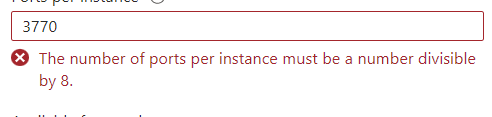# Is it possible to round a number to the nearest divisible by 8?

We need to set the outbound ports for standard load balancer on the basis of maximum number of backend instances.

For that we can use this formula

Number of IP * 64K/Number of backend instances

But after this, value has to be a nearest multiple of 8 as shown here. Is there anyway we can do this in terraform.Ignore… I have prepared the formula

floor(Number of IP * 64k/Number of instances)-(floor(Number of IP * 64k/Number of instances)%8)